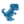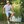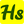0%
0%

# AC Voltmeter using Arduino

We are going to create an AC Voltage Measuring Device using Arduino, which will estimate the voltage of Alternating Current.

Similar projects worth following
2.6k views
Arduino AC Voltmeter will calculate the voltage of Alternating Current. This post reveals how to build a simple AC voltmeter that includes AC voltage with Arduino UNO board where voltage value is printed on 16×2 LCD.

Circuit Diagram

Working of Project

1. Step down voltage is obtained on the l.v side of the transformer which is fit to utilize across normal power rating resistors.

2. Then we receive specific voltage value across the 4.7k resistor.

3. Arduino utilizes this voltage as input from pin A0 in the form of analog values between 0 to 1023. 0 is considered as 0 volt and 1023 being 5v.

4. Arduino then changes this analog value into corresponding mains a.c. voltage by a formula.

n=(311/1023)*m volts or n=(m*.304177)

IoT Training Online will give a thorough view of IoT Applications.

• 1 × Power Managment IC, Transformer
• 1 × 1N4007 – High Voltage, High Current Rated Diode
• 1 × Capacitor 1 µF
• 1 × Zener Single Diode, 5.1 V
• 1 × Arduino UNO & Genuino UNO
• 1
Run a Code
```int m;  // initialise variable m
float n; //initialise variable n

void setup()
{
pinMode(A0,INPUT);  // set pin a0 as input pin  Serial.begin(9600);// begin serial
communication between Arduino and pc
}

void loop()
{
n=(m* .304177);    // converts analog value(x) into input ac supply value using this
formula
Serial.print("   analaog input  " ) ;   // specify name to the corresponding value to
be printed
Serial.print(m) ; // print input analog value on serial monitor
Serial.print("   ac voltage  ") ; // specify name to the corresponding value to be
printed
Serial.print(n) ; // prints the ac value on Serial monitor
Serial.println();
}```

Share

## Similar Projects

Project Owner Contributor

### DIY Arduino Miliohmmeter for measure low value resmircemk

Project Owner Contributor

### Digital Battery Operated PowersupplyThomas Van den Dries

Project Owner Contributor

### DIY simple Arduino Autorange capacitance metermircemk

Project Owner Contributor

### Arduino based simple Watt-meter shieldhalfstudents.in

# Does this project spark your interest?

Become a member to follow this project and never miss any updates